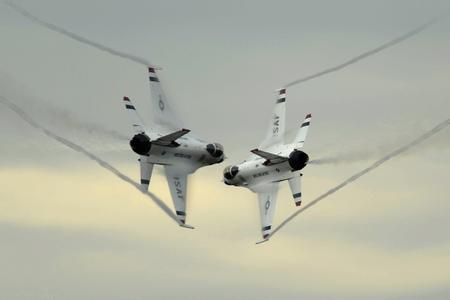# Pygame-GAME OVER```class Plane:

def restart(self):

self.x = 200

self.y = 600

def __init__(self):

self.restart()

def move(self):

x, y = pygame.mouse.get_pos()

x-= self.image.get_width() / 2

y-= self.image.get_height() / 2

self.x = x

self.y = y

plane = Plane()```

```def checkCrash(enemy, plane):

if (plane.x + 0.7*plane.image.get_width() > enemy.x) and (plane.x + 0.3*plane.image.get_width() < enemy.x + enemy.image.get_width()) and (plane.y + 0.7*plane.image.get_height() > enemy.y) and (plane.y + 0.3*plane.image.get_height() < enemy.y + enemy.image.get_height()):

return True

return False```

checkCrash把碰撞检测的结果用True或False返回。在游戏主循环里，我们增加一个记录游戏是否结束的变量gameover。把之前的游戏逻辑放在gameover为False的情况下。而当checkCrash为True时，就把gameover设为True。

g

```gameover = False

while True:

###

if not gameover:

###省略部分游戏逻辑

for e in enemies:

#如果撞上敌机，设gameover为True

if checkCrash(e, plane):

gameover = True

e.move()

screen.blit(e.image, (e.x, e.y))

#检测本体的运动

plane.move()

screen.blit(plane.image, (plane.x, plane.y))

else:

#待处理

pass```

THE END